Introduction to modvarsel

2018-11-05

Regression analysis studies the relationship between a $$p$$-dimensional covariable $$X=(X_1,\ldots,X_p)$$ and a numerical response variable $$Y$$. Three models are considered here:

• the linear parametric regression model : $$Y=\beta_0+\sum_{j=1}^p \beta_j X_j+\varepsilon$$, where $$\varepsilon$$ is a random error.
• the nonparametric regression model: $$Y=f(X)+\varepsilon$$.
• the single index semiparametric model: $$Y=f(\sum_{j=1}^p \beta_j X_j)+\varepsilon$$.

with the following estimations (regression) methods available in the package:

• multiple linear regression (linreg), principal component regression (pcr), partial least square regression (plsr), ridge regression (ridge) for parametric estimation,
• sliced inverse regression (sir) associated with kernel regression for semiparametric estimation,
• random forests regression (rf) for non parametric estimation.

The package modvarsel implements a computational methodology to choose among different regression methods the best one to predict the response variable $$Y$$ with a selection of $$p' \leq p$$ covariates strongly linked to $$Y$$. Usually the procedure of covariates selection is specific to the statistical method used to estimate the chosen model. Stepwise regression or lasso regression for instance select covariates in a parametric linear regression model. Here we use a generic methodology (working with any regression method) that simultaneously selects the best regression method and the most interesting covariates.

The package implements two main functions:

• the function choicemod implements an learning/test samples approach to determine which regression method (including covariates selection) is the most accurate.
• the function varimportance computes for each variable a measure of importance by estimating the response variable with some perturbations of the covariates and computing the error due to these perturbations.

The methodology applies to any regression dataset. It is illustrated here:

• on simulated data generated from a fictitious linear model where only some covariates are relevant to predict the response variable.
• on a real experimental dataset where meat tenderness is the response variable and muscular biomarkers are covariates.

Simulated data

Data have been simulated from a linear regression model without intercept $$Y=X^T\beta+\varepsilon$$ where $$X=(X_1,\ldots,X_p)$$ the $$p$$-dimensional covariate, $$Y$$ is the response variable and $$\varepsilon$$ is the random error term. Here

• the $$p$$=15 covariates $$X_j$$ are independant and follow a uniform distribution on $$[0 ; 0.7]$$,
• $$\varepsilon$$ is a standard normal error independant of $$X$$,
• $$\beta=(4,4,-3,-3,-2,0,\ldots,0)^T$$. Only the five first covariates as then relevant here to predict $$Y$$.

The R dataset simus (available in the package) contains $$n=200$$ observations of the covariates and the response variable generated with this model. More precisely a matrix $$\textbf{X}$$ of dimension $$200\times 15$$ contains the observations of the covariates and a vector $$\textbf{Y}$$ of size 200 contains the observations of the response variable.

library(modvarsel)
data(simus)
X <- simus$X # matrix of covariates Y <- simus$Y1 # response vector

Choice of the model

The aim of the function choicemod is to choose among several regression methods the best one to predict a response variable with an automatic selection of relevant covariates. In the package, the following regression methods are (for now) available:

• multiple linear regression (linreg),
• principal component regression (pcr),
• partial least square regression (plsr),
• ridge regression (ridge),
• sliced inverse regression (sir),
• random forests regression (rf).

The user selects a subset of regression methods and compares the mean square error (MSE) of each method (including covariates selection) given by the funcion choicemod. The function implements a computational learning/test approach to estimate the MSE values. The following procedure is repeats $$M$$ times:

• split randomly the data sample to build a learning sample and a test sample,
• with the learning sample and for each regression method:
• compute the importance of the $$p$$ covariates. The measure of importance of a variable is obtained by estimating the response variable with some random perturbations of this variable and computing the error due to these perturbations. If the variable has an effect on $$Y$$, the random permutation of its values in the sample will affect the estimation of $$Y$$ and its measure of importance will take a high value.
• select the $$p' \leq p$$ covariates with the highest measure of variable importance (VI). The number $$p'$$ is either defined by detecting a single change point position (in mean and variance) in the ordered VI values or by fixing a priori the number $$p'$$ of selected covariates.
• estimate both the complete model with the $$p$$ covariates and the reduced model with the $$p'$$ selected covariates.
• with the test sample and for each regression method, perform the mean square error (test MSE) of the estimated reduced model and the test MSE of the estimated complete model.

This procedure is replicated $$N$$ times giving $$N$$ values of test MSE for each regression method (with and without covariates selection). With this learning/test approach, models with different number of covariates can be compared, the one with the smaller test MSE being the best one. Parallel boxplots of test MSE (two boxplots per regression method) are then used to visually select the most relevant method.

Let us apply this procedure to compare multiple linear regression, sliced inverse regression and random forests regression to predict the response variable and select the five relevant covariates in the simulated regression dataset.

?choicemod
res <- choicemod(X, Y, method = c("linreg","sir","rf"), N = 50, nperm = 100)
# The computation time a bit long. So we use the results stored in the the dataset simus
res <- simus$res1 res is an object of class choicemod where results are stored in a list: • mse (resp. mse_all) is the matrix of the test MSE for each replication (in row) and each regression method (in column) for the reduced models (resp. the complete models), • sizemod is the matrix with the number of covariates selected in the reduced model for each replication (in row) and each regresion method (in column), • pvarsel is the matrix with the occurrences (in percent) of selection of each covariates (in row) and each regression method (in column). head(res$mse) # test MSE of the reduced models (6 first iterations over 50)
##      linreg       sir        rf
## 1 0.9491253 1.0728053 1.7220992
## 2 1.4489962 1.4775554 2.1127414
## 3 0.8262425 0.9335227 0.8906088
## 4 0.8241080 0.8743549 1.3355828
## 5 1.0966814 1.2867360 1.5966760
## 6 1.1407121 1.1670272 1.5703612
head(res$mse_all) # test MSE of the complete models (6 first iterations over 50) ## linreg_all sir_all rf_all ## 1 1.0009669 1.2017716 1.852430 ## 2 1.4829048 1.5119091 2.273554 ## 3 0.9274959 0.9848524 1.058876 ## 4 0.8972986 0.9961270 1.367208 ## 5 1.0982142 1.3259726 1.806408 ## 6 1.1700504 1.1725220 1.744519 Let us now describe the methods boxplot and barplot defined for the objects of class choicemod. Parallel boxplots of the MSE The parallel boxplots below are built with the MSE values obtained at each replication of the procedure implemented in choicemod (values in res$mse) for the reduced models (with covariates selection). These boxplots show that for the simulated data, the parametric method linreg and the semiparametric method sir outperform the non parametric method rf. This result is expected as the underlying simulation model is a parametric linear regression model.

?boxplot.choicemod
## Rendering development documentation for 'boxplot.choicemod'
boxplot(res, type = "reduced", col = rgb(0,0,1,0.2),
ylab = "test MSE",
main = "Models with variables selection, N=50")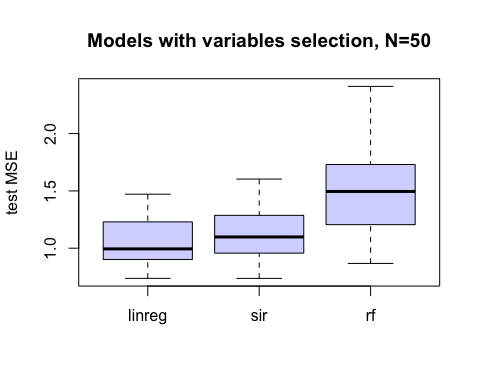This result remains true when complete models are used (no covariates selection).

boxplot(res,type="complete",col=rgb(0,0,1,0.2),
ylab = "test MSE",
main="Models without variables selection, N=50")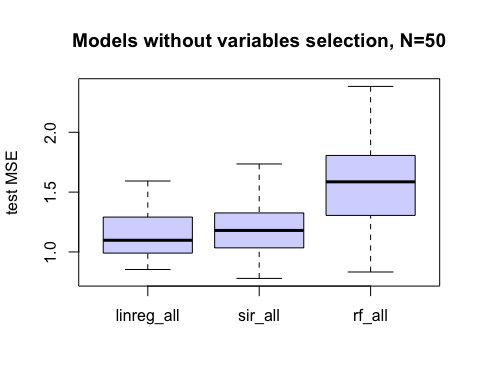Moreover we can see below that both linreg and sir are slighly better with covariate selection (two left boxplots) than without (two right boxplots). The selection of variables seems to improve here the mean square error of the two regression methods.

boxplot(res, method = c("linreg", "sir"),
type = "both", ylab = "test MSE",
col = rgb(0,1,0,0.2), las = 2,
main = "N = 50 replications")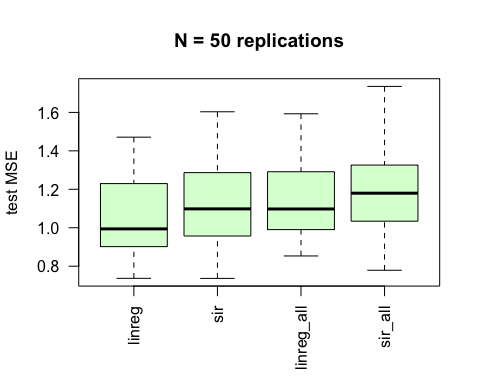Barplots of the selected covariates

The barplot below is built with the occurences (in percent) of selection of each covariates with the regression method linreg (values in the first column of res$pvarsel). This barplot shows that the proportion of selection of the 5 covariates $$X_1,\ldots,X_5$$ is equal to 1. It means that these variables have been been selected at each replication. ?barplot.choicemod ## Rendering development documentation for 'barplot.choicemod' barplot(res, method = "linreg", type = "varsel", las = 2, main = "linreg", col = rgb(1,1,0,0.2))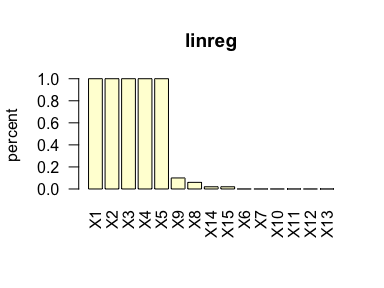The next barplot is built with the occurences (in percent) of the number of variables selected with the linreg method (values in the first column of res$sizemod). This barplot shows that a reduced model of size 5 has been selected more than 40 times (over $$M=50$$ replications).

barplot(res, method = "linreg", type = "sizemod", las = 2,
main = "linreg", col = rgb(0,1,1,0.2))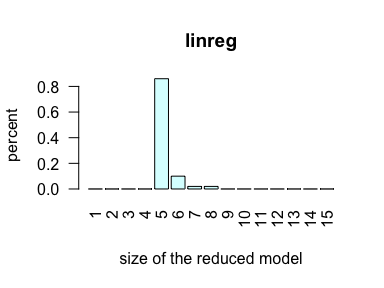To sum-up the five first covariates are important to predict the response variable and the other covariates are almost never selected. This result is expected because we know from the simulation scheme that the first five variables $$X_1,\ldots,X_5$$ are linked with $$Y$$.

The next barplots are obtained with the sir method. They show that sir selects more irrelevant variables (not linked with $$Y$$) than linreg.

barplot(res, method="sir", type="varsel", las = 2,
main = "sir", col = rgb(1,1,0,0.2))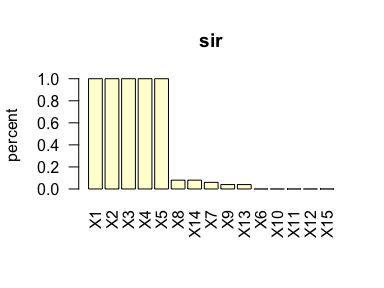barplot(res, method = "sir", type = "sizemod", las = 2,
main = "sir", col = rgb(0,1,1,0.2))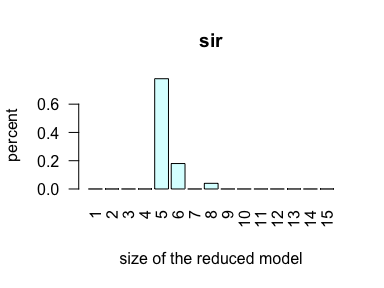Finally the most appropriate model seems to be the parametric linear regression model (as expected).

Identify and select useful covariates

The function varimportance computes for a given regression method (linreg, pcr, plsr, ridge, sir, rf) the importance of the $$p$$ covariates. Let us describe the general procedure. Let $$S={(x_i,y_i ),i=1,…,n}$$, be a sample of $$n$$ observations of the covariate $$X$$ and the response variable $$Y$$. The variable importance ($$VI$$) of a covariate $$X_j$$ is calculated as follows: $VI_j=\frac{1}{n} \sum_{i=1}^n (y_i-\hat{y_i}^{(j)})^2,$ where $$\hat{y_i}^{(j)}$$ is the prediction of the response variable when the observations of the $$j$$th covariate are randomly permuted in the sample $$S$$. If a covariate $$X_j$$ is important, the random permutation of its observations will affect the prediction of $$Y$$ and increase the error in $$VI_j$$. Covariates with higher $$VI$$ are then more important to predict the response variable. This procedure is replicated $$nperm$$ times ($$nperm$$ random permutations) giving $$nperm$$ values of $$VI$$ for each covariate.

Let us apply this procedure with the regression method linreg and $$nperm=100$$ replications to perform the importance of the $$p=15$$ covariates of the simulated dataset.

imp <- varimportance(X, Y, method = "linreg", nperm = 100)

imp is an object of class varimportance where results are stored in a list:

• mat_imp is the matrix of the $$VI$$ values for each replication (in row) and each covariate (un column).
• base_imp is the original mean square error.
imp$mat_imp[1:5,] # VI for the 5 first replications (over 100) ## X1 X2 X3 X4 X5 X6 X7 X8 X9 X10 X11 X12 X13 X14 X15 ## [1,] 1.5 1.4 1.4 1.3 1.1 0.96 0.96 0.97 0.96 0.96 0.94 0.97 0.95 0.97 0.92 ## [2,] 1.5 1.4 1.4 1.3 1.1 0.96 0.97 0.97 0.97 0.96 0.95 0.96 0.97 0.97 0.95 ## [3,] 1.5 1.4 1.4 1.3 1.1 0.96 0.96 0.97 0.97 0.96 0.96 0.97 0.97 0.97 0.97 ## [4,] 1.5 1.4 1.4 1.3 1.1 0.95 0.96 0.97 0.97 0.96 0.96 0.96 0.97 0.97 0.97 ## [5,] 1.5 1.4 1.4 1.3 1.1 0.96 0.97 0.92 0.96 0.95 0.96 0.96 0.97 0.97 0.96 colMeans(imp$mat_imp) # mean VI of the 15 covariates
##   X1   X2   X3   X4   X5   X6   X7   X8   X9  X10  X11  X12  X13  X14  X15
## 1.48 1.41 1.38 1.34 1.12 0.96 0.96 0.96 0.97 0.96 0.96 0.96 0.97 0.97 0.96
imp$base_imp #original MSE taken as baseline ##  0.96 The first 5 variables $$X_1,\ldots,X_5$$ have the highest mean $$VI$$ and are the only one with significantly greater $$VI$$ than the baseline MSE. Let us now describe the methods plot and select defined for the objects of class varimportance. Plots of the importance of the covariates The plot method provides two graphical representation: the parallel boxplots of the $$VI$$ of the covariates and the plot of the mean $$VI$$ of the covariates. The parallel boxplots below are built with the $$nperm=100$$ measures of $$VI$$ of the $$p=15$$ covariates of the simulated dataset. The values availables in imp$mat_imp.

?plot.varimportance
## Rendering development documentation for 'plot.varimportance'
plot(imp, choice = "boxplot", col = "lightgreen",
main = "linreg method and nperm=100 permutations")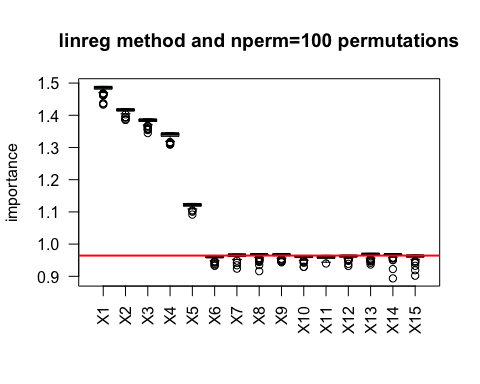The horizontal red line represents the original MSE (given in imp$vase_imp). The five variables $$X_1,\ldots,X_5$$ are obviously the only covariates clearly above this red line. The plot of the mean $$VI$$ of the covariates is given below. plot(imp, choice = "meanplot", main = "mean of nperm=100 permutations")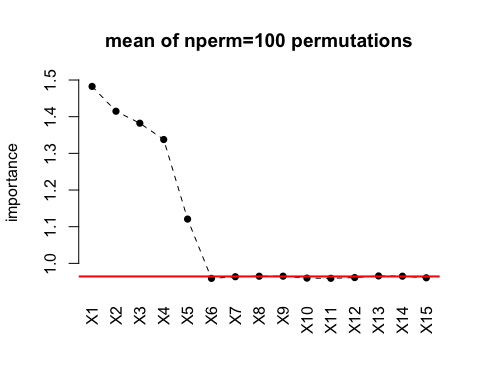It is possible to visualize the same plot (the mean $$VI$$) with the variables ordered by decreasing order of importance and with a vertical green line indicating a single change point position (in mean and variance). plot(imp, choice = "meanplot", order = TRUE, cutoff = TRUE, main = "ordered mean VI")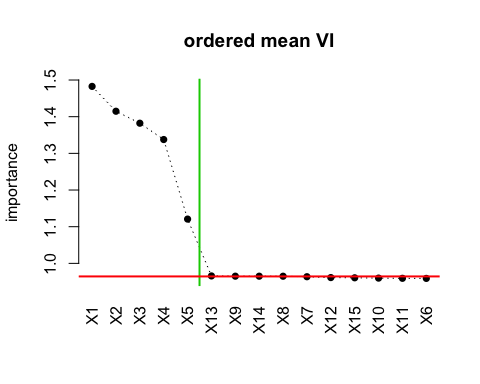The change point criterion (green line) selects the same 5 variables. Selection of the important covariates The method select chooses automaticaly $$p' \leq p$$ covariates relevant to predict the response variable $$Y$$. By default a change point criterion is used to define a cutoff value (green line on the plot above) and the covariates with mean $$VI$$ above this cutoff value are selected. ?select.varimportance ## Rendering development documentation for 'select.varimportance' select(imp, cutoff=TRUE) # by default ##$var
##  "X1" "X2" "X3" "X4" "X5"
##
## $indices ##  1 2 3 4 5 In that case the number of selected covariates is not controled. It is also possible to choose the number of covariates to select rather than using the changepoint cutoff value. For instance above the 7 covariates with the highest mean $$VI$$ are selected. select(imp, cutoff=FALSE, nbsel=7) ##$var
##  "X1"  "X2"  "X3"  "X4"  "X5"  "X13" "X9"
##
## $indices ##  1 2 3 4 5 13 9 Estimate the final model and make prediction Once a regression method is chosen, the user may want to predict new data. We provide here some R code • to estimate the final model (using $$p' \leq p$$ selected covariates) with a specific regression methods (linreg, sir or rf for instance), • to predict the response variable for new observations. The dataset simus contains a matrix of 10 new observations. Xnew <- simus$Xnew

We want now to predict the response variable for these 10 new observations. We suppose that the five first covariates have been selected whatever the regression method.

#sel <- select(imp, cutoff=TRUE)$indices sel <- 1:5 # matrices with the selected covariates X <- X[, sel] Xnew <- Xnew[, sel] Multiple linear regression The parametric linear regression model is estimated here by multiple linear regression. #estimate the linear model m1 <- lm(Y~.,data = data.frame(X)) Plot of the true values versus the predictions Ypred <- predict(m1,newdata = data.frame(X)) plot(Y,Ypred, main = "linreg") abline(0,1)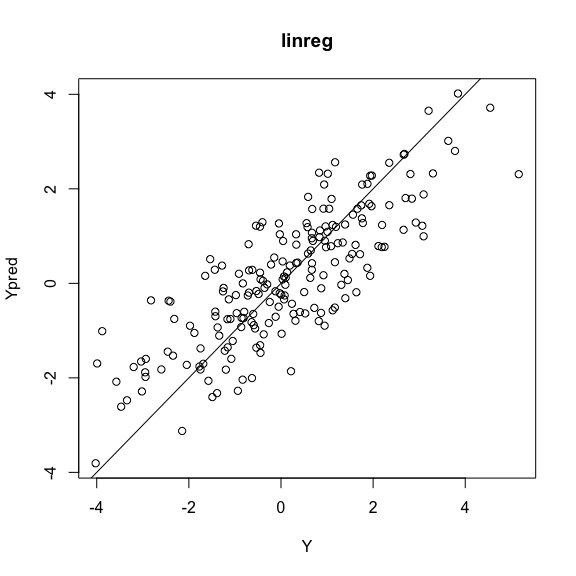Predictions of the new observations newpred1 <- predict(m1,newdata = data.frame(Xnew)) newpred1 ## 1 2 3 4 5 6 7 8 9 10 ## 0.21 0.43 -1.02 1.82 1.51 1.15 -2.54 -0.86 0.40 -0.76 Sliced inverse regression The single index semiparamtric model is estimated in two steps: • sliced inverse regression estimate the parameter $$\beta$$ of the index, • kernel regression estimates the nonparametric link function. The bandwidth for the kernel regression is tuned by LOO cross-validation. # estimate beta beta <- edrGraphicalTools::edr(Y, X, H = 10, K = 1, method = "SIR-I")$matEDR[, 1, drop = FALSE]
index <- X %*% beta

# tune the bandwidth
hopt <- cv_bandwidth(index, Y, graph.CV = FALSE)$hopt Plot of the true values versus the predictions Ypred <-ksmooth(index, Y, kernel = "normal", bandwidth = hopt, x.points = index)$y[rank(index)]
plot(Y,Ypred, main = "sir+kernel")
abline(0,1)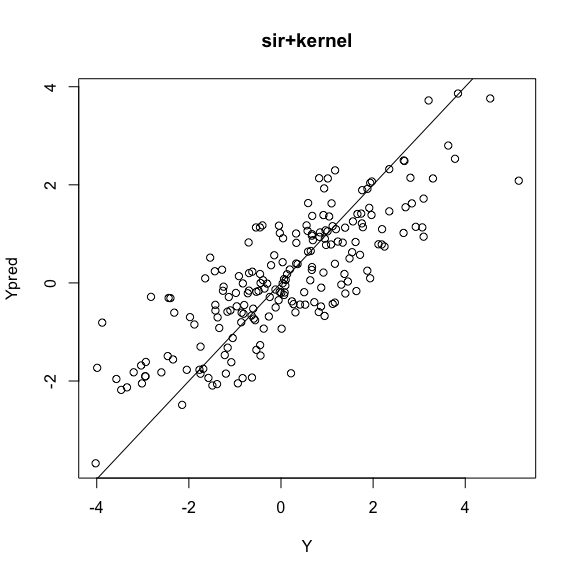Predictions of new data

indexnew <- Xnew%*%beta
newpred2 <- ksmooth(index, Y, kernel = "normal",
bandwidth = hopt,
x.points = indexnew)$y[rank(indexnew)] newpred2 ##  0.22 0.42 -0.88 1.64 1.31 1.08 -2.15 -0.64 0.34 -0.59 Random forests regression. The nonparametric model is estimated with random regression forests. The number of variables randomly sampled as candidates at each split is tuned with respect to Out-of-Baf error estimate. #tune mtry bestmtry <-randomForest::tuneRF(X, Y, trace = FALSE, plot =FALSE) ## Warning in rfout$mse/(var(y) * (n - 1)/n): Recycling array of length 1 in vector-array arithmetic is deprecated.
##   Use c() or as.vector() instead.

## Warning in rfout$mse/(var(y) * (n - 1)/n): Recycling array of length 1 in vector-array arithmetic is deprecated. ## Use c() or as.vector() instead. ## 0.065 0.05 ## Warning in rfout$mse/(var(y) * (n - 1)/n): Recycling array of length 1 in vector-array arithmetic is deprecated.
##   Use c() or as.vector() instead.
## 0.06 0.05
## Warning in rfout$mse/(var(y) * (n - 1)/n): Recycling array of length 1 in vector-array arithmetic is deprecated. ## Use c() or as.vector() instead. ## -0.022 0.05 #build the forest m3 <- randomForest::randomForest(X, Y, mtry = bestmtry) ## Warning in rfout$mse/(var(y) * (n - 1)/n): Recycling array of length 1 in vector-array arithmetic is deprecated.
##   Use c() or as.vector() instead.

Plot of the true values versus the predictions

Ypred <- predict(m3, newdata = X, type = "response")
plot(Y, Ypred, main = "random forest")
abline(0,1)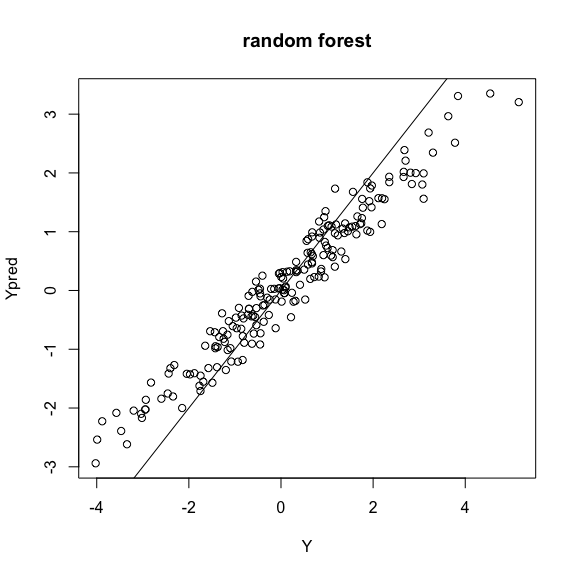We observe a strong overfitting here.

Predictions of new data

newpred3 <- predict(m3, newdata = Xnew, type = "response")
newpred3
##      1      2      3      4      5      6      7      8      9     10
## -0.321  0.184 -0.531  0.912  0.696  1.000 -1.528 -0.456  0.120 -0.015

Comparison of the three vectors of predictions

matplot(cbind(newpred1,newpred2,newpred3), ylab = "predictions",
xlab = "new observations",  cex=0.8)
legend("bottomleft", inset = 0.01, legend = c("linreg","sir","rf"), col = c(1:3),
pch = c("1","2","3"), cex=0.8)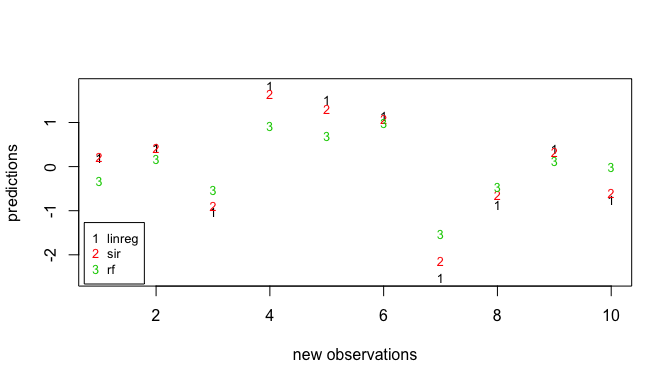Principal component regression

The linear regression model is estimated by principal component regression. the number of principal components. The number of principal components is tuned using 10-fold cross validation and a change point detection (in mean and variance) method.

# tune the number of components
m4 <- pls::pcr(Y~., data = as.data.frame(X),
validation="CV", scale=FALSE)
n <- nrow(X)
mse_cv <- m4$validation$PRESS/n
ncomp <- find.cpt(mse_cv)

Plot of the true values versus the predictions

Ypred <- predict(m4, newdata=X)[ , , ncomp]
plot(Y,Ypred, main = "pcr")
abline(0,1)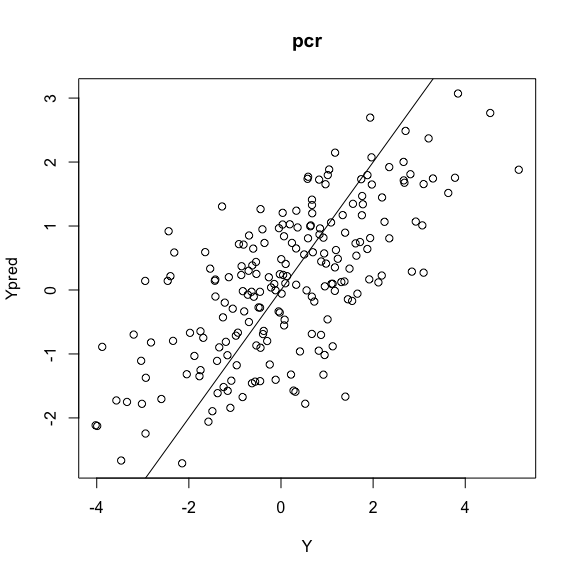Predictions of new data

newpred4 <- predict(m4, newdata=Xnew)[ , , ncomp]
newpred4
##   -1.16 -0.27 -1.01  0.70  0.89  0.23 -1.81 -0.69  0.44 -0.64

Partial least squares regression

The linear regression model is estimated by partial least squares regression (PLS). The number of PLS components is tuned using 10-fold cross validation and a change point detection (in mean and variance) method.

# tune the number of PLS components
m5 <- pls::plsr(Y~., data = as.data.frame(X),
validation="CV", scale=FALSE)
n <- nrow(X)
mse_cv <- m5$validation$PRESS/n
ncomp <- find.cpt(mse_cv)

Plot of the true values versus the predictions

Ypred <- predict(m5, newdata=X)[ , , ncomp]
plot(Y,Ypred, main = "plsr")
abline(0,1)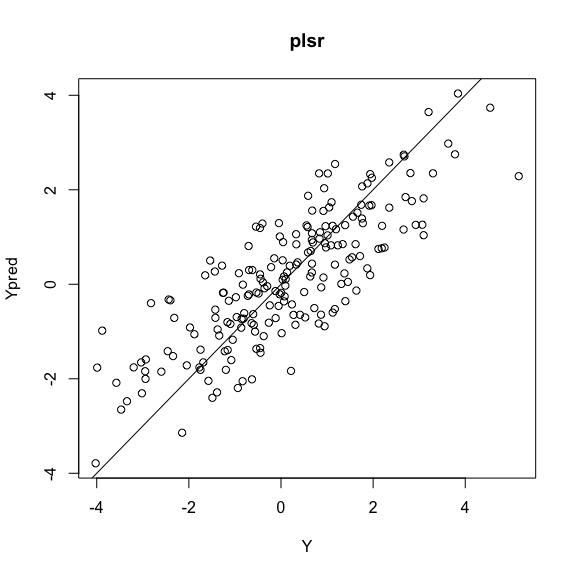Predictions of new data

newpred5 <- predict(m4, newdata=Xnew)[ , , ncomp]
newpred5
##   -0.84 -0.19  0.66  0.52  0.99  0.30 -1.36 -1.16  0.17  0.36

Ridge regression

The linear regression model is estimated by ridge regression. The regularization parameter $$\lambda$$ is tuned using 10-fold cross validation.

# tune the regularization parameter
m6 <- glmnet::cv.glmnet(X, Y,
family = "gaussian", alpha=0, standardize = FALSE,
nfolds = 10, grouped=FALSE)

Plot of the true values versus the predictions

Ypred <- predict(m6, X, s = "lambda.min")
plot(Y,Ypred, main = "ridge")
abline(0,1)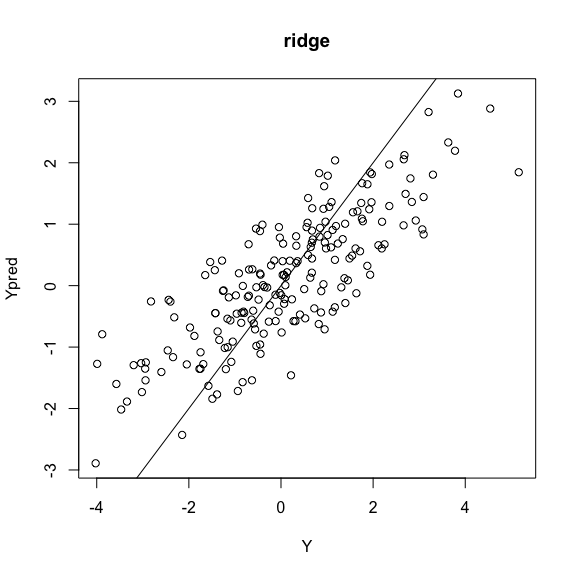Predictions of new data

newpred5 <- predict(m6, Xnew,  s = "lambda.min")
newpred5
##            1
##  [1,]  0.091
##  [2,]  0.310
##  [3,] -0.724
##  [4,]  1.401
##  [5,]  1.199
##  [6,]  0.893
##  [7,] -1.959
##  [8,] -0.693
##  [9,]  0.347
## [10,] -0.529

A real data example

We will now apply the same methodology (choice of a regression method including variables selection) with a real dataset. This dataset describes 71 young bulls on 21 muscular biomarkers and a numerical variable measuring meat tenderness. The data have been obtained on animals coming from the EU FP6 Integrated Project ProSafeBeef (FOODCT-2006-36241).The aim here is to select among the 21 muscular biomarkers, the most predictive of the toughness of the meat.

• The response variable (WB55a14J) is the warner-bratzler shear force measured on m. Semitendinosus (raw meat cooked at 55°C). It measures the toughness of the meat.
• The 21 biomarkers are listed here:

1. Metabolic enzymes:
• malate dehydrogenase (mdh1),
• $$\beta$$-enolase 3 (eno3),
• lactate dehydrogenase chain B (ldhb),
2. Heat shock proteins:
• $$\alpha\beta$$-crystallin (abcrysta),
• HSP20 (hsp20),
• HSP27 (hsp27),
• HSP40 (hsp40),
• HSP70-1A/B (hsp701b),
• HSP70/Grp75 (grp75),
• HSP70-8 (hsp708),
3. Oxidative proteins:
• protein deglycase (DJ1),
• peroxiredoxin6 (prdx6),
• superoxide dismutase (sod1),
4. Structural proteins:
• $$\alpha$$-actinin 2 (actine),
• MLC-1F (mlc1f),
• Myosin heavy chain-I (myhcI), -II (myhcII) and -IIx (myhcIIx),
• F-actin-capping protein subunit $$\beta$$ (capz),
• myosin binding protein H (mybpH),
5. Cell death, protein binding and proteolysis:
• $$\mu$$-calpain (microcal).

The R dataset psbst (available in the package) contains the data frame psbst$dat` with the description of the 71 bulls on the 21 biomarkers and the meat tenderness response variable. data("psbst") X <- psbst$dat[,-22] #  matrix of covariates (biomarkers)
Y <- psbst$dat$WB55a14J # response variable (meat tenderness)

Choice of the model

Let us first use the function choicemod to compare multiple linear regression, sliced inverse regression and random forest regression.

res <- choicemod(X, Y, method = c("linreg", "sir", "rf"),
N=50, nperm = 100)
# The computation time a bit long. So we use the results stored in the the dataset psbst
res <- psbst$res Boxplots to compare the regression methods boxplot(res, type = "both", main = "N = 50 replications", ylab = "test MSE", ylim=c(0,200), col = rgb(0,1,0,0.2), las = 2,)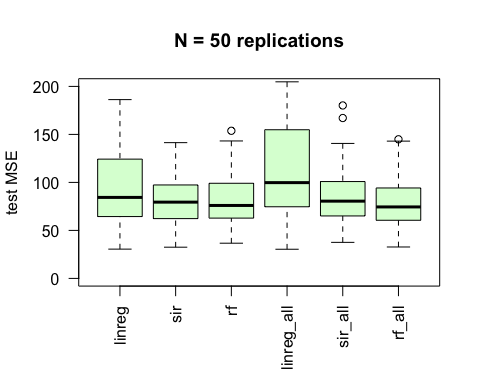The regression methods perform as well with covariates selection than without (three left boxplots compared to the three boxplots on the right) and no regression method seems to outperform clearly. Let us see if the three regression methods • select the same covariates, • select the same number of covariates. Barplots with informations about the selected covariates • With the linreg regression method barplot(res, method = "linreg", type = "varsel", main = "linreg", las = 2, col = rgb(1,1,0,0.2))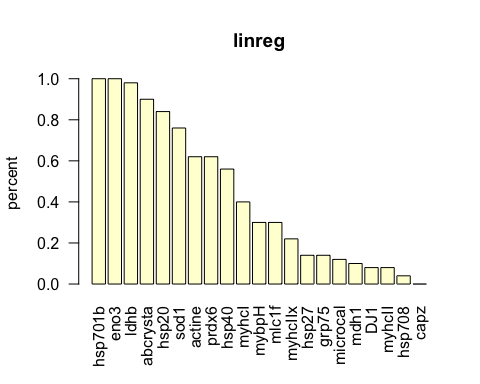barplot(res, method = "linreg", type = "sizemod", main = "linreg", las = 2, col = rgb(0,1,1,0.2))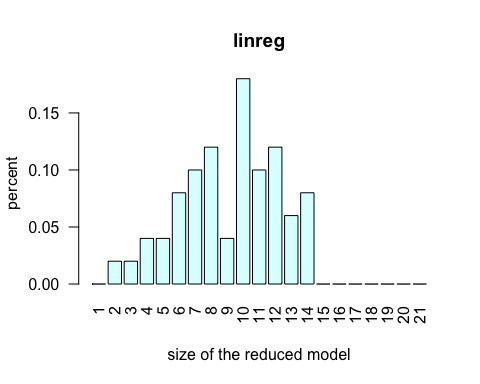• With the sir regression method barplot(res, method = "sir", type = "varsel", main = "sir", las = 2, col = rgb(1,1,0,0.2))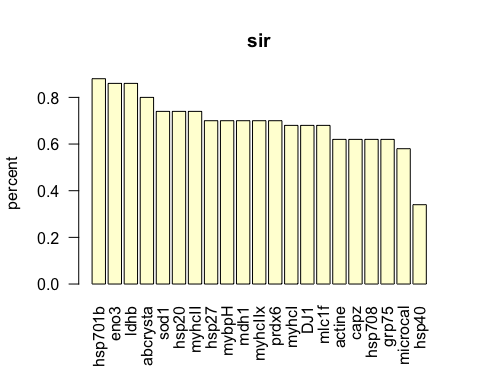barplot(res, method = "sir", type = "sizemod", main = "sir", las = 2, col = rgb(0,1,1,0.2))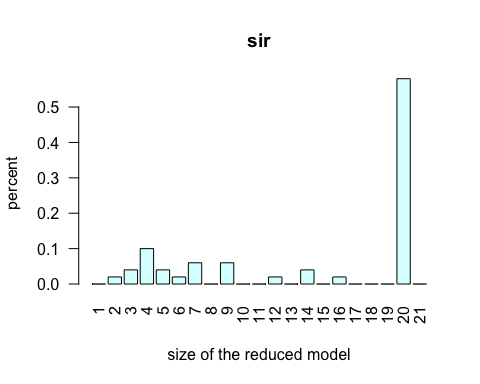• With the rf regression method barplot(res, method = "rf", type = "varsel", main = "rf", las = 2, col = rgb(1,1,0,0.2))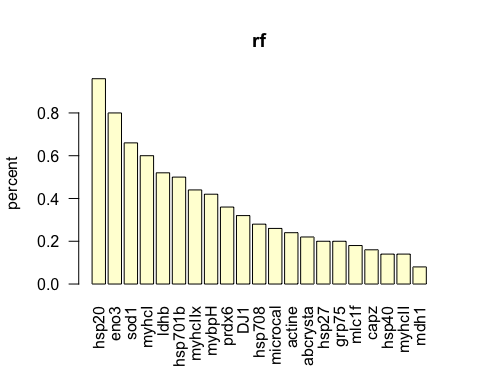barplot(res, method = "rf", type = "sizemod", main = "rf", las = 2, col = rgb(0,1,1,0.2))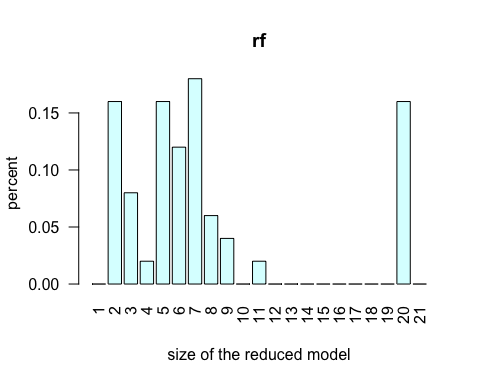To conclude the linreg method seems to be, for this dataset, a reasonable choice. The predictive quality of linreg is very comparable to that of sir and rf (see the parallel boxplots above) and the size of the reduced models are smaller (less biomarkers as covariates) (see the barplots above). Selection of important biomarkers The $$VI$$ of the 21 biomarkers are calculated with the varimportance function and the linreg regression method. imp1 <- varimportance(X,Y,method="linreg",nperm=200) # The computation time a bit long. So we use the results stored in the the dataset psbst imp1 <- psbst$imp1

The boxplot of the $$VI$$ values show that 10 biomarkers (green boxplot) are above the red line (the original baseline MSE).

colsel <- rep("white", 21)
colsel[c(2:8,12,17,20)] <- "lightgreen"
plot(imp1, choice = "boxplot",
main = "linreg", col = colsel)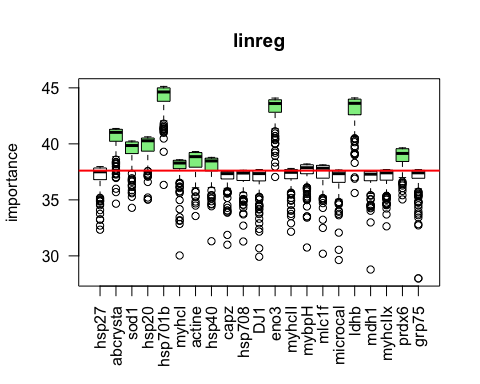The plot of the ordered mean $$VI$$ values shows that the cutoff value defined by detecting a single change point position (in mean and variance) (the horizontal green line) selects 2 more biomarkers.

plot(imp1, choice = "meanplot",
order = TRUE, cutoff = TRUE, main="linreg")